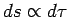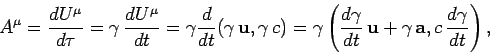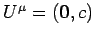Next: The current density 4-vector Up: Relativity and electromagnetism Previous: Proper time

## 4-velocity and 4-acceleration

We have seen that the quantitytransforms as a 4-vector under a general Lorentz transformation [see Eq. (1389)]. Sinceit follows that(1426)

also transforms as a 4-vector. This quantity is known as the 4-velocity. Likewise, the quantity(1427)

is a 4-vector, and is called the 4-acceleration.

For events along the world-line of a particle traveling with 3-velocity, we have(1428)

where use has been made of Eq. (1422). This gives the relationship between a particle's 3-velocity and its 4-velocity. The relationship between the 3-acceleration and the 4-acceleration is less straightforward. We have(1429)

whereis the 3-acceleration. In the rest frame of the particle,and. It follows that(1430)

(note thatis an invariant quantity). In other words, the 4-acceleration of a particle is always orthogonal to its 4-velocity.Next: The current density 4-vector Up: Relativity and electromagnetism Previous: Proper time
Richard Fitzpatrick 2006-02-02